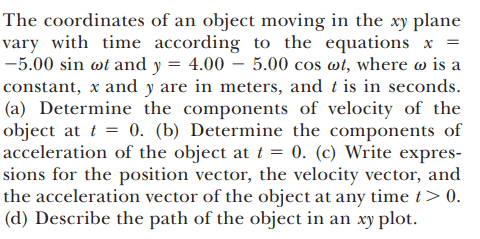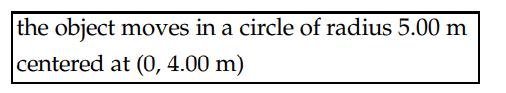# Describing path of the object in an xy plane

• ChiralSuperfields

#### ChiralSuperfields

Homework Statement
Relevant Equations
For part(d) of this problem,The solution is,However, how did they know that the object moves in a circle of radius 5.00m centered at (0,4.00m)?

Many thanks!

What is the equation of a circle centered at ##(x_0,y_0)##?

•ChiralSuperfields
The coordinates satisfy the equation of such a circle. This is how they know.

•ChiralSuperfields
Thank you for your replies @kuruman and @nasu!

The equation of a circle centered at ##(x_0, y_0)## is ##(x - x_0)^2 + (y - y_0)^2 = r^2##

Many thanks!

Can you bring what you have in that form? Remember that ##~\sin^2x+\cos^2x=1.##

•ChiralSuperfields
Can you bring what you have in that form? Remember that ##~\sin^2x+\cos^2x=1.##

Here is what I got:

##x^2 + y^2 = r^2 ##
##(-5.00sin\omega t)^2 + (4.00 - 5cos\omega t)^2 = r^2 ##
##25sin^2\omega t + 16 - 40cos\omega t +25cos^2\omega t = r^2 ##
##25(sin^2\omega t + cos^2\omega t) +16 - 40cos\omega t = r^2 ##
## 41 - 40cos\omega t = r^2 ##

EDIT: I'm now thinking that I should put the individual position function in the x-components in the y-components in the circle formula I posted.

Many thanks!

Here is what I got:
Don't try to prove that way ...
EDIT: I'm now thinking that I should put the individual position function in the x-components in the y-components in the circle formula I posted.
Yes.
We know that ##x=-5sinωt## and ##y-4=-5cosωt## so we have:
##x^2+(y-4)^2=25sin^2ωt+25cos^2ωt=25## and we know that ##x^2+(y-4)^2=5^2## is equation of a circle with ##r=5## that is centered at ##(0,4)##.

•ChiralSuperfields
Don't try to prove that way ...

Yes.
We know that ##x=-5sinωt## and ##y-4=-5cosωt## so we have:
##x^2+(y-4)^2=25sin^2ωt+25cos^2ωt=25## and we know that ##x^2+(y-4)^2=5^2## is equation of a circle with ##r=5## that is centered at ##(0,4)##.
•